# Forty

Forty five of the 80 students were girls. What is the ratio of girls to boys?

r =  9:7

### Step-by-step explanation:Did you find an error or inaccuracy? Feel free to write us. Thank you!Tips to related online calculators
Need help to calculate sum, simplify or multiply fractions? Try our fraction calculator.
Check out our ratio calculator.

## Related math problems and questions:

• The ratioThe ratio of girls to boys in Mrs. White's class is 3:2.  If there are 12 boys, how many girls are in the classroom?
• 80 students80 students were asked what type of test they preferred. 50 students said they liked multiple choice and 42 liked true or false. If 36 liked both multiple choice and true or false types, how many students preferred multiple choice only?
• Exhibition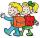The teacher paid 280 Kč for 4.A students for admission to the exhibition. How many students were at the exhibition?
• Pupils 7There are 40 pupils in a certain class. 3/5 of the class are boys. How many are girls?
• StudentsThere are 2/6 girls out of 1050 students at the school. Of these, 4/5 have another sibling. How many girls are in school? How many boys are in school? How many girls do not have a sibling?
• Boys to girlsThe ratio of boys to girls at a party is 3:5. If six more boys arrived and four girls left the party, the ratio of boys to girls would be 5:6. How many people were at the party initially?
• Brian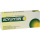Brian divides 80 by 7. Between what two whole numbers is his answer?
• The classroom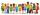In the seventh class the number of boys and girls are at a ratio of 4:5. Boys are 16. How many total students are in the classroom?
• English and math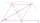1/5 of a class study English, and 3/5 study mathematics. If the class consist of 80 students, how many students study English and mathematics in the class?
• Report 2A School reports students to teacher ratio of 6:1. If there are 45 teachers in the School, how many students are there?
• GroupsIn the 6th class there are 60 girls and 72 boys. We want to divide them into groups so that the number of girls and boys is the same. How many groups can you create? How many girls will be in the group?
• Summer camp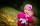84 children spent their holidays at the summer camp. There were 8 fewer boys than girls. What percentage were boys and what percentage were girls?
• Chicks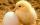How many chicks were hatched from 4500 eggs, when an average of 100 eggs hatched 87 chicks?
• Passenger boatTwo-fifths of the passengers in the passenger boat were boys. 1/3 of them were girls and the rest were adult. If there were 60 passengers in the boat, how many more boys than adult were there?
• Ratio of countsThere are 15 boys and 13 girls in the class. What are the ratio of boys and girls?
• A class IV.CIn a class 2/5 were boys. 30 were girls. How many more girls than boys are there?
• Twelve eggs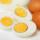A dozen eggs cost 80 cents . how much will 3½ dozen eggs cost?